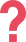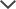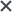I used the Wonderlic personality prep pack. Your preparatory questions tuned my mind in a correct way to face the real questions.
Josh
I got the NNAT pack. I like the variety of questions offered in the test pack, as they provided us with an idea as to what will be on the test.
Musleha
I got the Wonderlic Personality Cognitive prep and it was money well spent!
Maknev
The fact that the practice tests were online and very visual was great, as it helped my kids to be more responsive to exercises.
Morgan
Very good explanations! I love it and I learnt many things from you... thanks for such a great help!
Abigail
We used the OLSAT Level D tests. The content and layout are excellent and the site is so easy to use. Our son's results were excellent and we would definitely recommend this site to other parents.
Ben
I got the Wonderlic BST prep. Everything was exactly as on TestPrep-Online's practice tests. If you do well on TestPrep-Online, you will do well on the actual test...the practice tests were in perfect alignment with the actual test.
Debbie

## About the CLEP College Pre-Calculus Exam

Our CLEP College Pre-Calculus product consists of two full-length simulations of the real exam. The CLEP Pre-Calculus is divided into two sections. In the first section, you are allowed a calculator and are required to solve 25 questions in 50 minutes. The second section forbids the use of calculators and consists of 23 questions to be solved in 40 minutes.

Within these two sections, you will face two kinds of math problem: routine, straightforward math problems, and non-routine, more complicated math problems. The latter type of math question will require you to have a strong grasp of mathematical concepts and be able to apply these concepts and your mathematical skills to answer the questions.

Sound complicated? It doesn’t have to be. Early preparation can help you face this section of the test with the confidence and knowledge required to pass.

You will be required to answer 48 questions in 90 minutes, and you may use a calculator to help you in one of the sections.

## Breakdown of CLEP Pre-Calculus Topics

The CLEP Pre-Calculus test is divided into two sections. However, there is a distribution of the number of questions from each of the topics that appear in the sections. Below you will see a detailed description of the topics in the CLEP Pre-Calculus test along with the number of questions in total for each topic.

Algebraic Expressions, Equations and Inequalities - 20% of the test (approximately 9 questions).
Students are expected to:

• Solve equations and inequalities. This includes linear, quadratic, rational, radical, exponential, logarithmic, polynomial, trigonometric, with absolute value.
• Perform operations on algebraic expressions.
• Solve systems of equations (linear and nonlinear).

Functions: Concept, Properties and Operations - 15% of the test (approximately 7 or 8 questions).
In this section, the students are expected to:

• Demonstrate an understanding of a function (the definition), general properties of functions (domain, range, and others), function notation.
• Perform symbolic operations with functions (evaluating, finding inverse functions, a combination of both, finding zeroes of functions, etc.).

Representations of Functions: Symbolic, Graphical and Tabular - 30% of the test (approximately 15 questions).
Students are tested in their ability to:

• Recognize and perform operations and transformations on functions presented symbolically, graphically or in the forms above.
• Demonstrate an understanding of basic properties of functions
• Recognize elementary functions. This includes all of the following: linear, quadratic, absolute value, square root, polynomial, rational, exponential, logarithmic, trigonometric, inverse trigonometric and piecewise-defined functions (represented in any of the forms above).

Analytic Geometry - 10% of the test (approximately 4 or 5 questions)
This topic tests the if the students are able to demonstrate an understanding of the analytic geometry of lines, circles, parabolas, ellipses and hyperbolas.

Functions as Models
- 10% of the test (approximately 4 or 5 questions.)
This portion requires interpreting and constructing functions as models in order to translate ideas between symbolic, graphical, tabular, and verbal representations of functions.

Trigonometry and Its Applications
- 15% of the test (approximately 7 questions).
The CLEP Pre-Calculus measures your ability to demonstrate an understanding of basic trigonometric functions and their inverses. In addition to this, it measures your ability to apply the basic trigonometric ratios and identities (in right triangles and on the unit circle) and to apply trigonometry in different problem-solving contexts.

An important thing to note is that trigonometry is involved in most of the major topics and, as a result, accounts for more than 15 percent of the exam. Roughly speaking, between 30 to 40 percent of the exam questions either involve some knowledge in trigonometry, trigonometric functions, their properties, or similar.

## CLEP College Pre-Calculus Prep

Our simulations are just like the real exam – you will have 90 minutes to answer 48 questions.

Make sure to start studying two or three weeks in advance to properly understand all the topics. Each question contains thorough explanations with intuitive solutions for you to learn about the topics in the most comfortable way. You can retake all of our tests and track your progress. If you are having difficulty with one of the questions, try taking a break and then approaching the question again. It is also very useful to rewrite the explanations in your own words to check that you have truly understood the solution.

We will also provide CLEP Pre-Calculus study guides to help you better understand the concepts behind the questions.

CLEP and other trademarks are the property of their respective trademark holders. None of the trademark holders are affiliated with TestPrep-online or this website.

Need HelpNeed Help